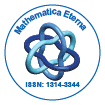# Mathematica EternaOpen Access

ISSN: 1314-3344

## Abstract

### On The Solutions of Linear Matrix Quaternionic Equations and Their Systems

Ahmet ÃÂIpek and Cennet Bolat CÃÂ¸ imen

In this paper, our main aim is to investigate the solvability, existence of unique solution, closed-form solutions of some linear matrix quaternion equations with one unknown and of their systems with two unknowns. By means of the arithmetic operations on matrix quaternions, the linear matrix quaternion equations that is considered herein could be converted into four classical real linear equations, the solutions of the linear matrix quaternion equations are derived by solving four classical real linear equations based on the inverses and generalized inverses of matrices. Also, efficiency and accuracy of the presented method are shown by several examples.

Top